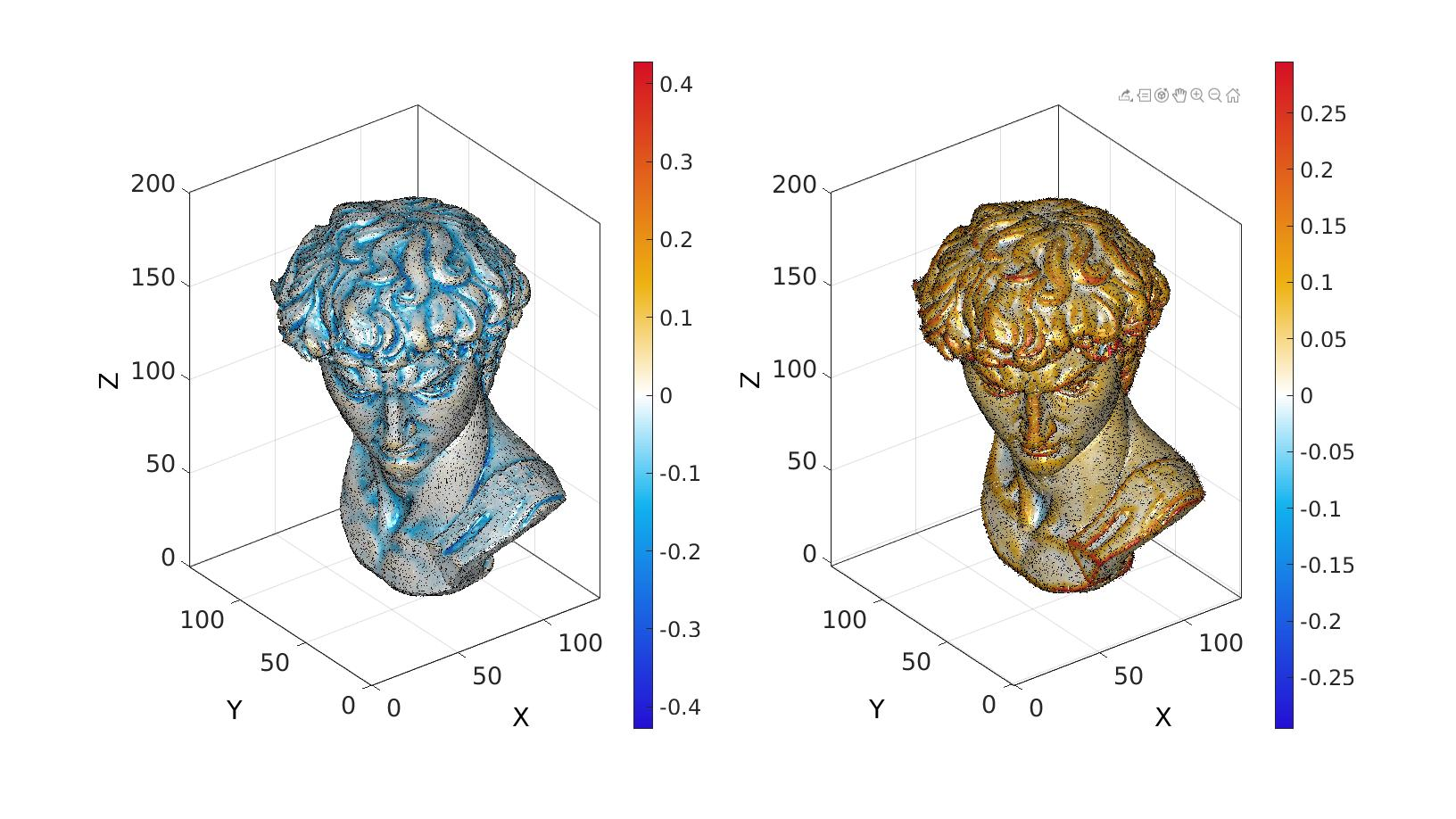# patchCurvature

Below is a demonstration of the features of the patchCurvature function

## Syntax

[Vd,Fd,Fds]=patchCurvature(V,F);

## Description

Computes curvature metrics for the patch data defined by the faces F and the vertices V.

## Examples

```clear; close all; clc;
```

Plot settings

```cMap=warmcold(250);
```
```[F,V]=graphicsModels(9);
% [F,V]=stanford_bunny;
```

## Compute curvature

```[U_min,U_max,C_min,C_max,C_mean,C_gauss] = patchCurvature(F,V);
```

## Visualize curvature on mesh

```% Compute plot variables
C_min_V=faceToVertexMeasure(F,V,C_min); %Vertex data for interpolated shading
C_max_V=faceToVertexMeasure(F,V,C_max); %Vertex data for interpolated shading
VN=patchCentre(F,V); %Element centres used for vector origins
vecPlotSize=mean(patchEdgeLengths(F,V)); %Vector plotting size

% Visualize
cFigure;
subplot(1,2,1); hold on;
hp=gpatch(F,V,C_min_V,'none',0.9);
hp.FaceColor='interp';
colormap(gca,cMap); colorbar;
quiverVec(VN,U_min,vecPlotSize,'k');
axisGeom;
c=max(abs(C_min(:)));
caxis(0.25*[-c c]);

subplot(1,2,2); hold on;
hp=gpatch(F,V,C_max_V,'none',0.9);
hp.FaceColor='interp';
quiverVec(VN,U_max,vecPlotSize,'k');
colormap(gca,cMap); colorbar;
axisGeom;
c=max(abs(C_max(:)));
caxis(0.25*[-c c]);

drawnow;
```GIBBON www.gibboncode.org

Kevin Mattheus Moerman, [email protected]

GIBBON footer text

GIBBON: The Geometry and Image-based Bioengineering add-On. A toolbox for image segmentation, image-based modeling, meshing, and finite element analysis.

Copyright (C) 2019 Kevin Mattheus Moerman

This program is free software: you can redistribute it and/or modify it under the terms of the GNU General Public License as published by the Free Software Foundation, either version 3 of the License, or (at your option) any later version.

This program is distributed in the hope that it will be useful, but WITHOUT ANY WARRANTY; without even the implied warranty of MERCHANTABILITY or FITNESS FOR A PARTICULAR PURPOSE. See the GNU General Public License for more details.

You should have received a copy of the GNU General Public License along with this program. If not, see http://www.gnu.org/licenses/.